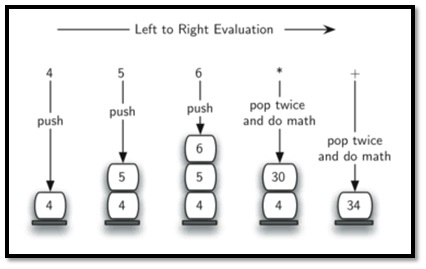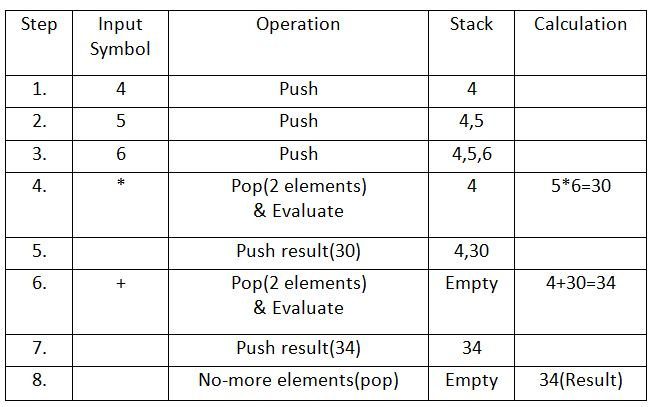# Evaluation of Postfix Expressions Using Stack [with C program]

Learn: How to evaluate postfix expression using stack in C language program? This article explains the basic idea, algorithm (with systematic diagram and table) and program to evaluate postfix expression using stack.
Submitted by Abhishek Jain, on June 19, 2017

As discussed in Infix To Postfix Conversion Using Stack, the compiler finds it convenient to evaluate an expression in its postfix form. The virtues of postfix form include elimination of parentheses which signify priority of evaluation and the elimination of the need to observe rules of hierarchy, precedence and associativity during evaluation of the expression.

As Postfix expression is without parenthesis and can be evaluated as two operands and an operator at a time, this becomes easier for the compiler and the computer to handle.

Evaluation rule of a Postfix Expression states:

1. While reading the expression from left to right, push the element in the stack if it is an operand.
2. Pop the two operands from the stack, if the element is an operator and then evaluate it.
3. Push back the result of the evaluation. Repeat it till the end of the expression.

### Algorithm

1) Add ) to postfix expression.
2) Read postfix expression Left to Right until ) encountered
3) If operand is encountered, push it onto Stack
[End If]
4) If operator is encountered, Pop two elements
i) A -> Top element
ii) B-> Next to Top element
iii) Evaluate B operator A
push B operator A onto Stack
5) Set result = pop
6) END

Let's see an example to better understand the algorithm:

Expression: 456*+Result: 34

## Evaluation of Postfix Expressions Using Stack

```/* This program is for evaluation of postfix expression
* This program assume that there are only four operators
* (*, /, +, -) in an expression and operand is single digit only
* Further this program does not do any error handling e.g.
* it does not check that entered postfix expression is valid
* or not.
* */

#include <stdio.h>
#include <ctype.h>

#define MAXSTACK 100 /* for max size of stack */
#define POSTFIXSIZE 100 /* define max number of charcters in postfix expression */

/* declare stack and its top pointer to be used during postfix expression
evaluation*/
int stack[MAXSTACK];
int top = -1; /* because array index in C begins at 0 */
/* can be do this initialization somewhere else */

/* define push operation */
void push(int item)
{

if (top >= MAXSTACK - 1) {
printf("stack over flow");
return;
}
else {
top = top + 1;
stack[top] = item;
}
}

/* define pop operation */
int pop()
{
int item;
if (top < 0) {
printf("stack under flow");
}
else {
item = stack[top];
top = top - 1;
return item;
}
}

/* define function that is used to input postfix expression and to evaluate it */
void EvalPostfix(char postfix[])
{

int i;
char ch;
int val;
int A, B;

/* evaluate postfix expression */
for (i = 0; postfix[i] != ')'; i++) {
ch = postfix[i];
if (isdigit(ch)) {
/* we saw an operand,push the digit onto stack
ch - '0' is used for getting digit rather than ASCII code of digit */
push(ch - '0');
}
else if (ch == '+' || ch == '-' || ch == '*' || ch == '/') {
/* we saw an operator
* pop top element A and next-to-top elemnet B
* from stack and compute B operator A
*/
A = pop();
B = pop();

switch (ch) /* ch is an operator */
{
case '*':
val = B * A;
break;

case '/':
val = B / A;
break;

case '+':
val = B + A;
break;

case '-':
val = B - A;
break;
}

/* push the value obtained above onto the stack */
push(val);
}
}
printf(" \n Result of expression evaluation : %d \n", pop());
}

int main()
{

int i;

/* declare character array to store postfix expression */
char postfix[POSTFIXSIZE];
printf("ASSUMPTION: There are only four operators(*, /, +, -) in an expression and operand is single digit only.\n");
printf(" \nEnter postfix expression,\npress right parenthesis ')' for end expression : ");

/* take input of postfix expression from user */

for (i = 0; i <= POSTFIXSIZE - 1; i++) {
scanf("%c", &postfix[i]);

if (postfix[i] == ')') /* is there any way to eliminate this if */
{
break;
} /* and break statement */
}

/* call function to evaluate postfix expression */

EvalPostfix(postfix);

return 0;
}
```

Output

```First Run:
Enter postfix expression, press right parenthesis ')' for end expression : 456*+)
Result of expression evaluation : 34

Second Run:
Enter postfix expression, press right parenthesis ')' for end expression: 12345*+*+)
Result of expression evaluation: 47
```

Languages: » C » C++ » C++ STL » Java » Data Structure » C#.Net » Android » Kotlin » SQL
Web Technologies: » PHP » Python » JavaScript » CSS » Ajax » Node.js » Web programming/HTML
Solved programs: » C » C++ » DS » Java » C#
Aptitude que. & ans.: » C » C++ » Java » DBMS
Interview que. & ans.: » C » Embedded C » Java » SEO » HR
CS Subjects: » CS Basics » O.S. » Networks » DBMS » Embedded Systems » Cloud Computing
» Machine learning » CS Organizations » Linux » DOS
More: » Articles » Puzzles » News/Updates